# Global views company profile

13. Nov 2017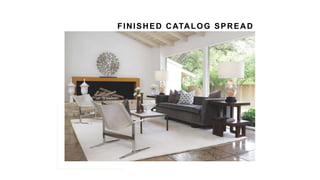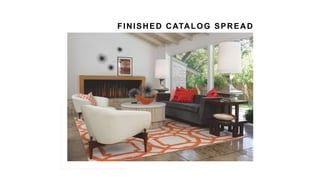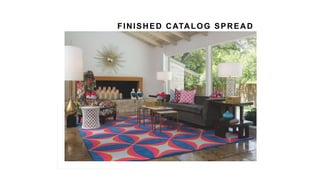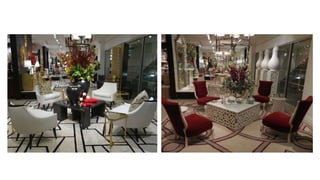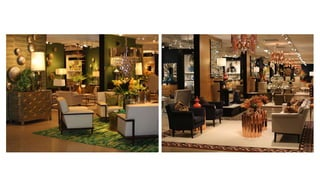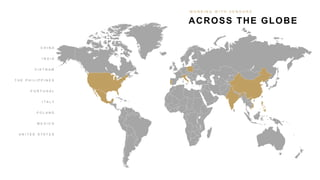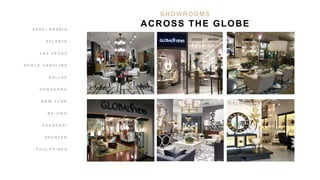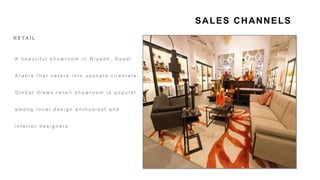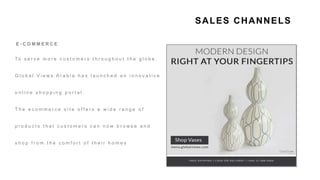1 von 34

### Global views company profile

• 2. W E A R E COMMITTED
• 3. M A R K E T I N G C R E AT I V E D I R E C T I O N WE ARE COMMITTED TO H E R E A R E A F E W T H I N G S B R A N D S TAT U S Q U A L I T Y & S TA N D A R D S G R O W T H S A L E S T O O L S V E N D O R R E L AT I O N S H I P S I N V E N T O RY & S H I P M E N T S
• 4. Global Views and Studio A Home are two of the leading brands in the home furnishings industry. W E ’ R E C O M M I T T E D T O MAINTAINING BRAND’S TOP-TIER STATUS WORLDWIDE
• 7. W E ’ R E C O M M I T T E D T O REQUIRING A HIGH STANDARD OF EXCELLENCE We push for the highest standards when it comes to quality, new product, trends, and overall direction.
• 11. W E ’ R E C O M M I T T E D T O INTRODUCING A MINIMUM OF 500 NEW ITEMS EACH YEAR Business grows as our offerings grow. Every year, we offer 500+ new product introductions between both brands.
• 13. W E ’ R E C O M M I T T E D T O PRODUCING TWO BEAUTIFUL CATALOGS EACH YEAR We distribute comprehensive catalogs for both Global Views and Studio A Home each January, as well as a condensed catalog each June.
• 14. THE CATALOG PROCESS 1. LAYOU TS 2. PH OTO SH OOT 3. ED ITIN G & PR IN TIN G A L O O K A T
• 18. We share creative and visual direction with our partners that can be interpreted and utilized throughout all showrooms. W E ’ R E C O M M I T T E D T O PROVIDING VISUAL MERCHANDISING DIRECTION
• 21. AN ONGOING MARKETING PROGRAM Instagram | Snachat | Twitter Facebook | LinkedIn W E ’ R E C O M M I T T E D T O
• 22. W E ’ R E C O M M I T T E D T O ANALYZING INVENTORY & PRIORITIZING SHIPMENTS Our buying team prioritizes shipments to our partners worldwide. We constantly analyze inventory needs to ensure all partners are in a healthy inventory position.
• 24. W e a r e c o m m i t t e d t o BUILDING & STRENGTHENING VENDOR RELATIONSHIPS We value the strong relationships we’ve built with factories producing our goods worldwide. We will continue to seek out new & unique vendors.
• 25. ACROSS THE GLOBE W O R K I N G W I T H V E N D O R S C H I N A I N D I A V I E T N A M T H E P H I L I P P I N E S P O R T U G A L I T A L Y P O L A N D M E X I C O U N I T E D S T A T E S
• 26. SHOWROOMS We are committed to serve customers: Exceptional Customer Service Value for Money Customer Care
• 27. S A U D I A R A B I A A T L A N T A L A S V E G A S N O R T H C A R O L I N A D A L L A S H O N G K O N G N E W Y O R K B E I J I N G S H A N G H A I S H E N Z E N P H I L I P P I N E S S H O W R O O M S ACROSS THE GLOBE
• 28. SHOWROOMS We are committed to serve customers: Exceptional Customer Service Value for Money Customer Care
• 29. SALES CHANNELS R E TA I L A b e a u t i f u l s h o w r o o m i n R i y a d h , S a u d i A r a b i a t h a t c a t e r s i n t o u p s c a l e c l i e n t e l e . G l o b a l V i e w s r e t a i l s h o w r o o m i s p o p u l a r a m o n g l o c a l d e s i g n e n t h u s i a s t a n d i n t e r i o r d e s i g n e r s
• 30. SALES CHANNELS E - C O M M E R C E To s e r v e m o r e c u s t o m e r s t h r o u g h o u t t h e g l o b e , G l o b a l V i e w s A r a b i a h a s l a u n c h e d a n i n n o v a t i v e o n l i n e s h o p p i n g p o r t a l . T h e e c o m m e r c e s i t e o f f e r s a w i d e r a n g e o f p r o d u c t s t h a t c u s t o m e r s c a n n o w b r o w s e a n d s h o p f r o m t h e c o m f o r t o f t h e i r h o m e s
• 31. SALES CHANNELS D E S I G N P R O J E C T S G l o b a l V i e w s R i y a d h o f f e r s d e s i g n c o n s u l t a t i o n s e r v i c e s f o r c l i e n t s i n t e r e s t e d i n g e t t i n g t h e i r h o m e / o f f i c e s d e s i g n e d b y o u r i n - h o u s e i n t e r i o r d e s i g n e r
• 32. SALES CHANNELS B 2 B G l o b a l V i e w s S a u d i A r a b i a a t t r a c t s w h o l e s a l e b u y e r s a c r o s s M i d d l e E a s t a n d N o r t h A f r i c a . F r o m e s t a b l i s h e d m u l t i - s t o r e c h a i n s t o w i d e r a n g e o f c l i e n t s H O S P I TA L I T Y G l o b a l V i e w s S a u d i A r a b i a w o r k s w i t h h o s p i t a l i t y p r o j e c t s o f v a r i o u s s i z e s i n M i d d l e E a s t a n d N o r t h A f r i c a
• 33. MIDDLE EAST AND NORTH AFRICA CLIENTS S A U D I A R A B I A A r w a D e s i g n s B o n H o m e P i e c e s S h o w r o o m A I n t e r i o r s U N I T E D A R A B E M I R AT E S N a k k a s h G a l l e r y B l o o m i n g d a l e s E l d i a r L E B A N O N M - S q u a r e B A H R A I N C a s t i l l o I n t e r i o r s J O R D A N H i g h H i l l I n v e s t m e n t s